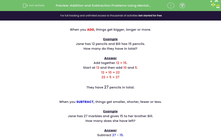# Use Mental Methods of Addition and Subtraction to Solve Word Problems

In this worksheet, students will solve problems based on addition or subtraction of tangible objects, quantities and measures.Key stage:  KS 1

Curriculum topic:   Number: Addition and Subtraction

Curriculum subtopic:   Understand Order for Addition/Subtraction

Difficulty level:#### Worksheet Overview

When you ADD, things get bigger, longer or more.Example

Jane has 12 pencils and Bill has 15 pencils.

How many do they have in total?

The easiest way to do this is to split the second number into tens and ones. If we split 15, we get 1 ten and 5 ones.

Start at 12 and add 10:   12 + 10 = 22

Then add on the 5 ones: 22 + 5 = 27

They have 27 pencils in total.

When you SUBTRACT, things get smaller, shorter, fewer or less.Example

Jane has 27 marbles and gives 15 to her brother Bill.

How many does she have left?

Subtract: 27 - 15.

Once again, split the second number into tens and ones. 15 is 1 ten and 5 ones.

Start at 27 and take away 10: 27 - 10 = 17

Then take away the 5 ones: 17 - 5 = 12

Jane has 12 left.

Are you ready to have a go at some questions?### What is EdPlace?

We're your National Curriculum aligned online education content provider helping each child succeed in English, maths and science from year 1 to GCSE. With an EdPlace account you’ll be able to track and measure progress, helping each child achieve their best. We build confidence and attainment by personalising each child’s learning at a level that suits them.

Get started